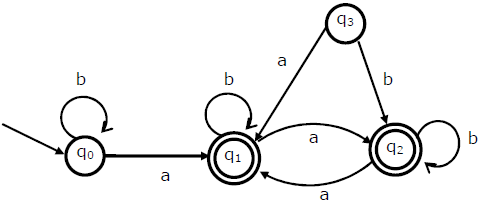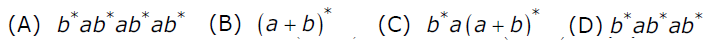Related Articles
GATE | GATE-CS-2007 | Question 74
• Difficulty Level : Hard
• Last Updated : 26 Feb, 2014

Consider the following Finite State Automaton. The language accepted by this automaton is given by the regular expression(A) A
(B) B
(C) C
(D) D

Explanation: In this case, we would at least have to reach q1 so that our string gets accepted. So, b* a is the smallest accepted string.
Now, at q1, any string with any number of a’s and b’s would be accepted. So, we append (a + b)* to the smallest accepted string.

Thus, the string accepted by the FSA is b* a (a + b)*.

Thus, C is the correct choice.

Please comment below if you find anything wrong in the above post.

Quiz of this Question

My Personal Notes arrow_drop_up